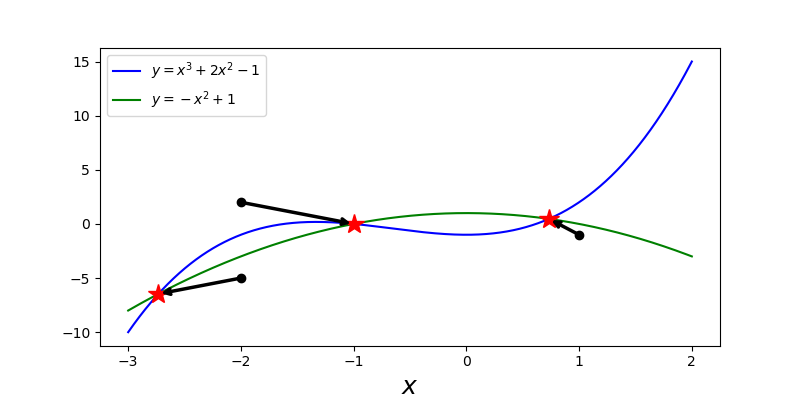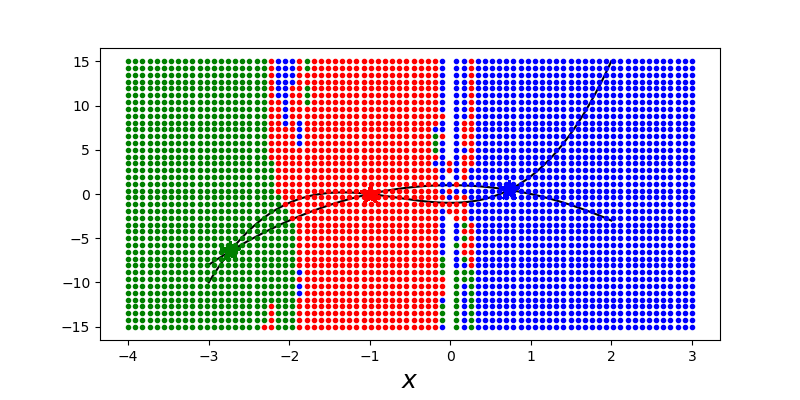## 线性方程组

### Square Systems

m = n 的 square systems 是一个重要的特例。它对应于方程数等于未知变量数的情况，因此它可能有一个唯一解。

$A$ 满秩时，可以保证解存在。但是，可能不能精确计算解。矩阵的条件数 $cond(A)$ 给出了衡量一个线性方程组好坏的条件。如果条件数接近 1，则说系统条件良置（well conditioned，条件数为 1 是理想条件），如果条件数很大，则系统条件病态（ill conditioned）。一个病态的线性方程组的解可能有很大误差。可以从一个简单的错误分析中获得关于条件数的直观解释。假设我们有一个形式为 $Ax=b$ 的线性方程组，其中 $x$ 是解向量。现在考虑 $b$ 的一个小变化，$\delta b$，由 $A(x+\delta x)=b+\delta b$ 给出解的相应变化 $\delta x$。由于方程的线性，我们有 $A\delta x = \delta b$。现在要考虑的一个重要问题是：与 $b$ 的变化相比，$x$ 的变化大吗？数学上我们可以用这些向量的范数之比来表述这个问题。具体而言，我们比较 $\lVert \delta x \rVert / \lVert x \rVert$$\lVert \delta b \rVert / \lVert b \rVert$，其中 $\lVert x \rVert$ 表示 $x$ 的范数。使用矩阵范数关系 $\lVert Ax \rVert \le \lVert A \rVert \cdot \lVert x \rVert$，我们可以写为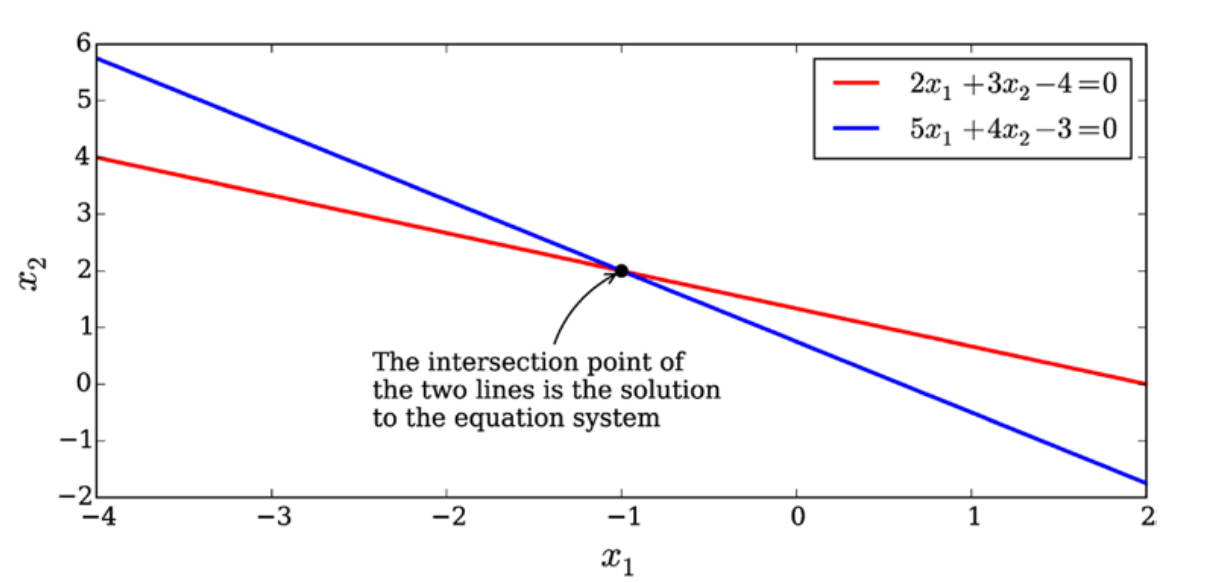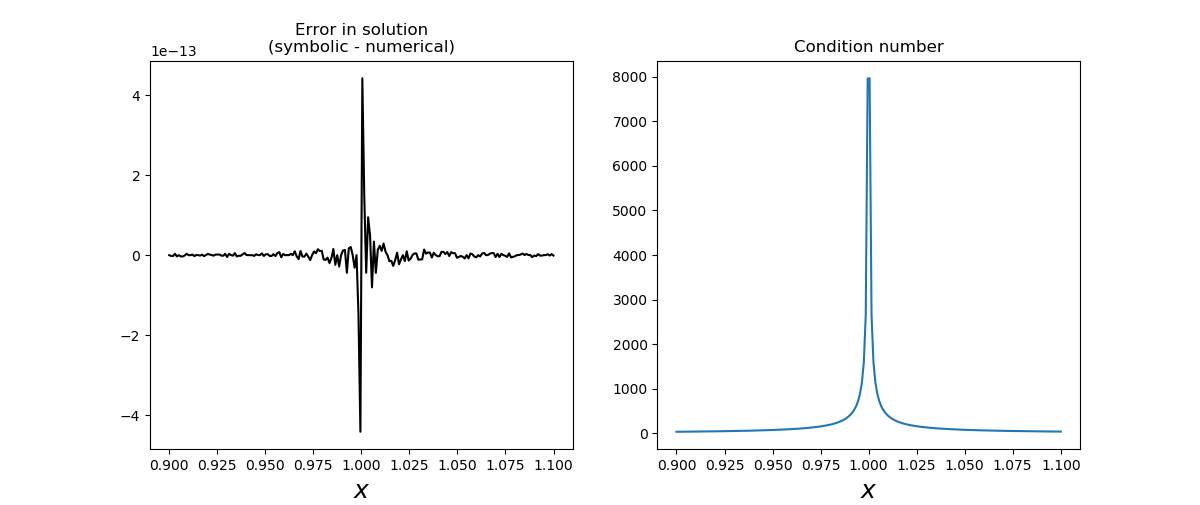### Rectangular Systems## 特征值问题

SymPy 和 SciPy 中的线性代数包都包含求解特征值问题的方法。在 SymPy 中，可以使用 Matrix 类的 eigenvals 和 eigenvects 方法，它们能够计算一些符号表达式元素的矩阵的特征值和特征向量。

## 非线性方程

### 单变量方程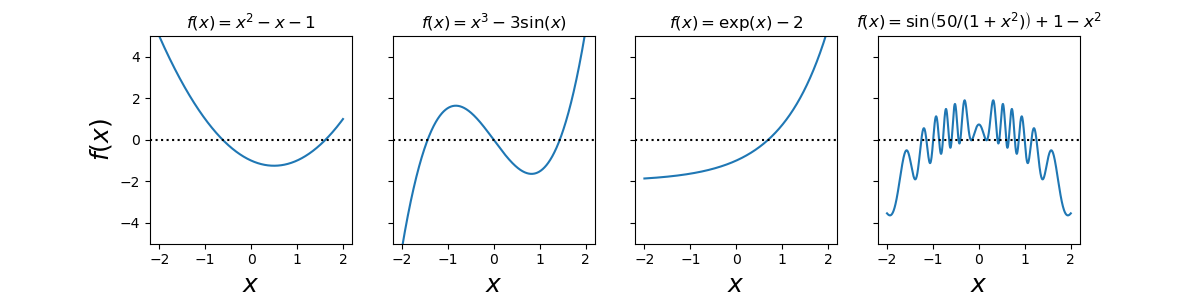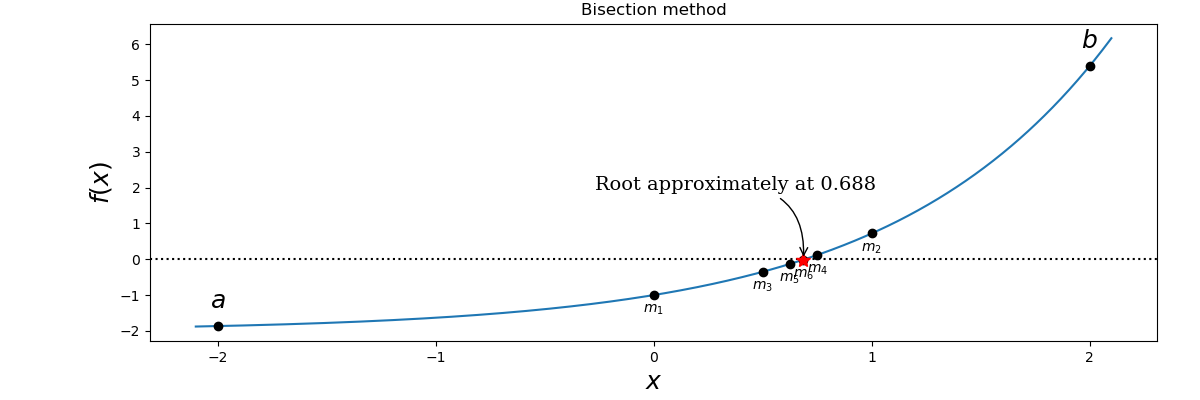SciPy 优化模块提供了多种数值求根函数。optimize.bisectoptimize.newton 函数实现了二分法和牛顿法的变体。optimize.bisect 有三个参数：第一个是 Pytho n函数（如 lambda 函数），它表示需要求根的数学函数，第二个和第三个参数是执行二分法的时间间隔。请注意，如前所述，函数的符号必须在 a 和 b 处不同，才能使用二分法。使用 optimize.bisect 函数，我们可以计算方程 $exp(x) -2 = 0$ 的根，

SciPy 优化模块为求根提供了额外的函数。具体而言，optimize.brentqoptimize.brenth 函数是二分法的变体，也能在函数符号改变的区间工作。 optimize.brentq 函数通常被认为是 SciPy 中首选的全方位求根函数。

### 非线性方程组

optimize.broyden1optimize.broyden2 可以用类似的方式使用。要指定 optimize.fsolve 的雅可比矩阵，我们需要定义一个函数来计算给定输入向量的雅可比行列式。这里我们可以使用 SymPy 推导：